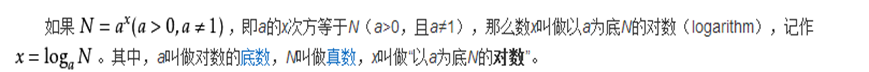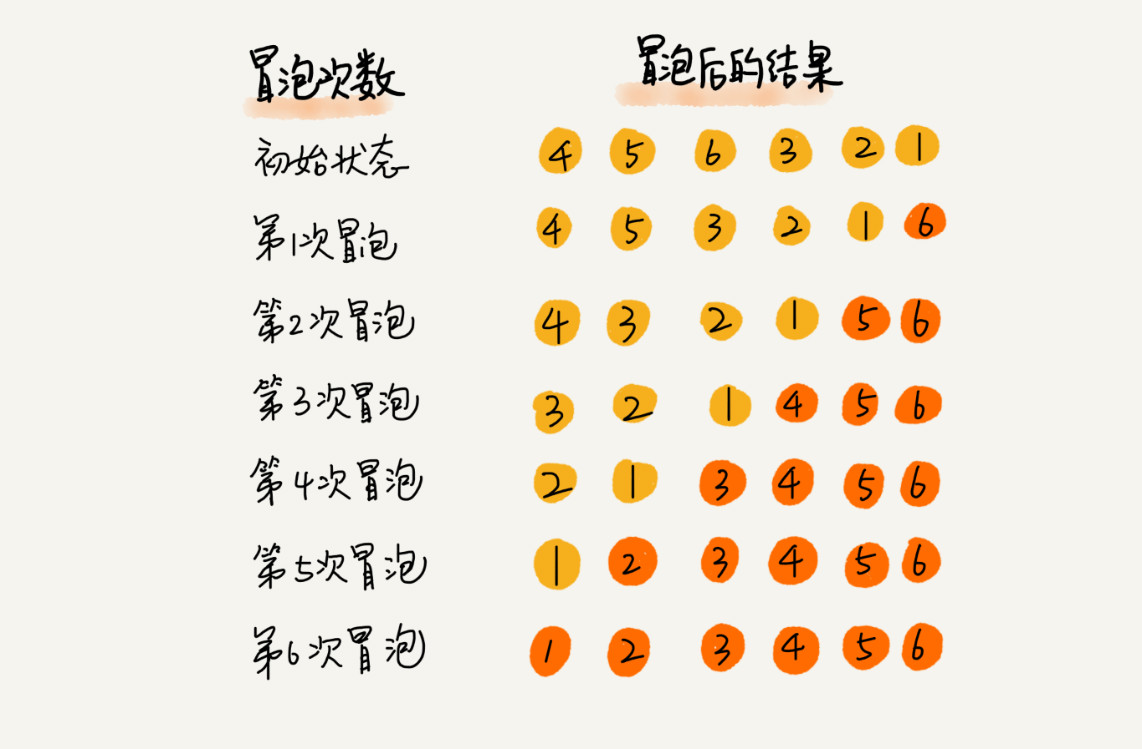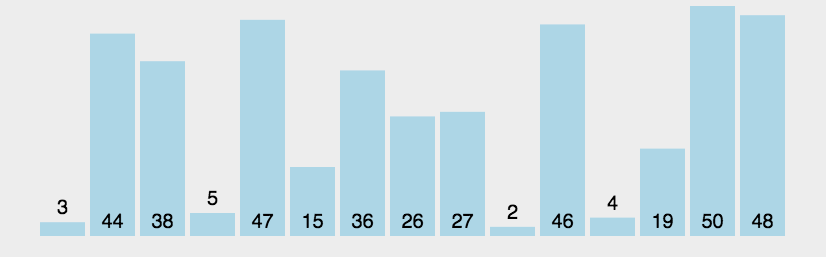# 新星计划 Day10【数据结构与算法】 排序算法

• 2022 年 7 月 18 日
• 本文字数：2793 字

阅读完需：约 9 分钟

# 新星计划 Day10【数据结构与算法】 排序算法

👩‍💻博客主页：京与旧铺的博客主页

✨欢迎关注🖱点赞🎀收藏⭐留言✒

🔮本文由京与旧铺原创，csdn 首发！

😘系列专栏：java 学习

👕参考网课：尚硅谷

💻首发时间：🎞2022 年 5 月 11 日🎠

🎨你做三四月的事，八九月就会有答案，一起加油吧

🀄如果觉得博主的文章还不错的话，请三连支持一下博主哦

🎧最后的话，作者是一个新人，在很多方面还做的不好，欢迎大佬指正，一起学习哦，冲冲冲

[TOC]

# 👜50.排序算法介绍和分类

• 内部排序：指将需要处理的所有数据都加载到内部存储器中进行排序。

• 外部排序法：数据量过大，无法全部加载到内存中，需要借助外部存储进行排序。

• 算法的时间复杂度：

• 度量一个程序(算法)执行时间的两种方法

• 事后统计的方法

• 这种方法可行, 但是有两个问题：

• 一是要想对设计的算法的运行性能进行评测，需要实际运行该程序

• 二是所得时间的统计量依赖于计算机的硬件、软件等环境因素

• 这种方式，要在同一台计算机的相同状态下运行，才能比较那个算法速度更快

• 事前估算的方法 通过分析某个算法的时间复杂度来判断哪个算法更优

# 👛51.时间频度介绍和特点

• 2n+20 和 2n 随着 n 变大，执行曲线无限接近，20 可以忽略

• 3n+10 和 3n 随着 n 变大，执行曲线无限接近，10 可以忽略

• 2n^2+3n+10 和 2n^2 随着 n 变大，执行曲线无限接近，可以忽略 3n+10

• n^2+5n+20 和 n^2 随着 n 变大，执行曲线无限接近，可以忽略 5n+20

• 随着 n 值变大，5n^2+7n 和 3n^2 + 2n ，执行曲线重合，说明 这种情况下，5 和 3 可以忽略

• 而 n^3+5n 和 6n^3+4n ，执行曲线分离，说明多少次方式关键

# 🧤52.时间复杂度计算和举例说明

• 一般情况下，算法中的基本操作语句的重复执行次数是问题规模 n 的某个函数，用 T(n)表示，若有某个辅助函数 f(n)，使得当 n 趋近于无穷大时，T(n) / f(n) 的极限值为不等于零的常数，则称 f(n)是 T(n)的同数量级函数。记作 T(n)=Ｏ( f(n) )，称Ｏ( f(n) ) 为算法的渐进时间复杂度，简称时间复杂度。

• T(n) 不同，但时间复杂度可能相同。 如：T(n)=n²+7n+6 与 T(n)=3n²+2n+2 它们的 T(n) 不同，但时间复杂度相同，都为 O(n²)。

• 计算时间复杂度的方法：

• 用常数 1 代替运行时间中的所有加法常数 T(n)=n²+7n+6 => T(n)=n²+7n+1

• 修改后的运行次数函数中，只保留最高阶项 T(n)=n²+7n+1 => T(n) = n²

• 去除最高阶项的系数 T(n) = n² => T(n) = n² => O(n²)

• 常数阶 O(1)

• 对数阶 O(log2n)

• 线性阶 O(n)

• 线性对数阶 O(nlog2n)

• 平方阶 O(n^2)

• 立方阶 O(n^3)

• k 次方阶 O(n^k)

• 指数阶 O(2^n)

### 常数阶O(1)

• 无论代码执行了多少行，只要是没有循环等复杂结构，那这个代码的时间复杂度就都是 O(1)

int i = 1;int j = 2;++i;j++;int m = i + j;复制到剪贴板复制失败复制成功

• 上述代码在执行的时候，它消耗的时候并不随着某个变量的增长而增长，那么无论这类代码有多长，即使有几万几十万行，都可以用 O(1)来表示它的时间复杂度。

### 对数阶O(log2n)

int n = 100;int i = 1;while (i < n) {    i = i * 2;}复制到剪贴板复制失败复制成功### 线性阶O(n)

int n = 100;int j = 0;for (int i = 1; i <= n; i++) {    j = i;    j++;}复制到剪贴板复制失败复制成功

### 线性对数阶O(nlogN)

int n = 100;int m;int i;for (m = 1; m < n; m++) {    i = 1;    while (i < n) {        i = i * 2;    }}复制到剪贴板复制失败复制成功

### 平方阶O(n²)

int x;int j;int i = 0;int n = 100;for (x = 1; i <= n; x++) {    for (i = 1; i <= n; i++) {        j = i;        j ++;    }}复制到剪贴板复制失败复制成功

# 🩱53.平均和最坏时间复杂度介绍

### 平均时间复杂度和最坏时间复杂度

• 平均时间复杂度是指所有可能的输入实例均以等概率出现的情况下，该算法的运行时间。

• 最坏情况下的时间复杂度称最坏时间复杂度。一般讨论的时间复杂度均是最坏情况下的时间复杂度。 这样做的原因是：最坏情况下的时间复杂度是算法在任何输入

• 实例上运行时间的界限，这就保证了算法的运行时间不会比最坏情况更长。平均时间复杂度和最坏时间复杂度是否一致，和算法有关

## 空间复杂度

• 类似于时间复杂度的讨论，一个算法的空间复杂度(Space Complexity)定义为该算法所耗费的存储空间，它也是问题规模 n 的函数

• 空间复杂度(Space Complexity)是对一个算法在运行过程中临时占用存储空间大小的量度。有的算法需要占用的临时工作单元数与解决问题的规模 n 有关，它随着 n 的增大而增大，当 n 较大时，将占用较多的存储单元，例如快速排序和归并排序算法就属于这种情况

• 在做算法分析时，主要讨论的是时间复杂度。从用户使用体验上看，更看重的程序执行的速度。一些缓存产品(redis、memcache)和算法(基数排序)本质就是用空间换时间

# 🎍54.冒泡排序算法图解

## 冒泡排序

### 基本介绍

• 冒泡排序（Bubble Sorting）的基本思想是：通过对待排序序列从前向后（从下标较小的元素开始），依次比较相邻元素的值，若发现逆序则交换，使值较大的元素逐渐从前移向后部，就象水底下的气泡一样逐渐向上冒。

• 因为排序的过程中，各元素不断接近自己的位置，如果一趟比较下来没有进行过交换，就说明序列有序，因此要在排序过程中设置一个标志 flag 判断元素是否进行过交换。从而减少不必要的比较。(这里说的优化，可以在冒泡排序写好后，在进行)

• 遍历 1 次过后，可以指定已经排好序数据的下标 flag，那么下 1 次排序排到 flag 就可以了，flag 不断向前移动，移动到第 1 位之后排序就结束了## 评论